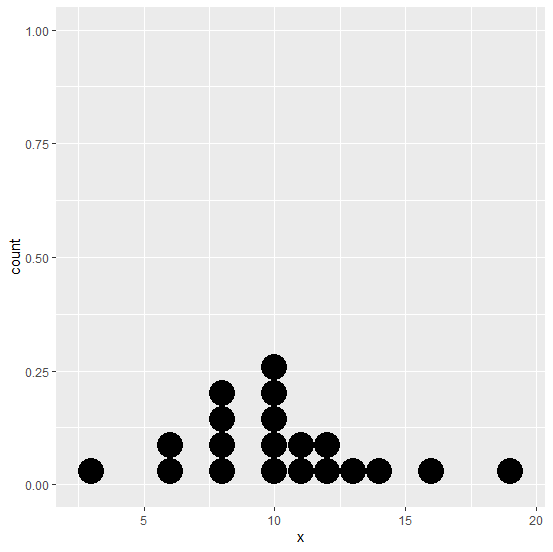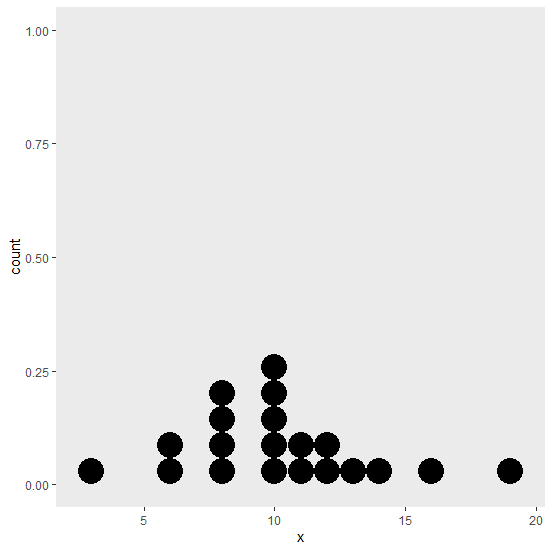# How to create a dotchart using ggplot2 without gridlines in R?

To create a dotchart using ggplot2 in R, we can use geom_dotplot function but the default gridlines will be in the output. If we want to remove the gridlines from the plot then theme function can be added in the rest of the command as theme(panel.grid=element_blank()).

## Example

Live Demo

Consider the below data frame −

set.seed(214)
x<−rpois(20,10)
df<−data.frame(x)
df

## Output

  x
1 8
2 10
3 13
4 16
5 10
6 11
7 12
8 11
9 3
10 8
11 10
12 12
13 10
14 6
15 8
16 6
17 19
18 10
19 8
20 14

library(ggplot2)
ggplot(df,aes(x))+geom_dotplot(binwidth=1)

## OutputCreating the dotchart without gridlines −

## Example

ggplot(df,aes(x))+geom_dotplot(binwidth=1)+theme(panel.grid=element_blank())

## Output